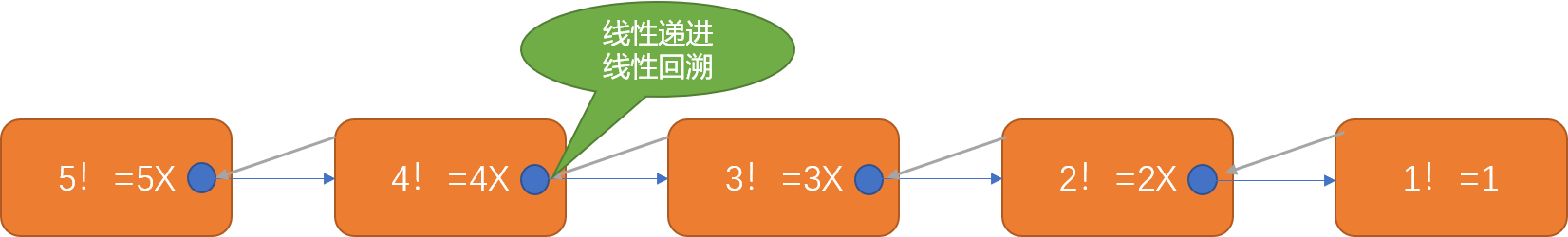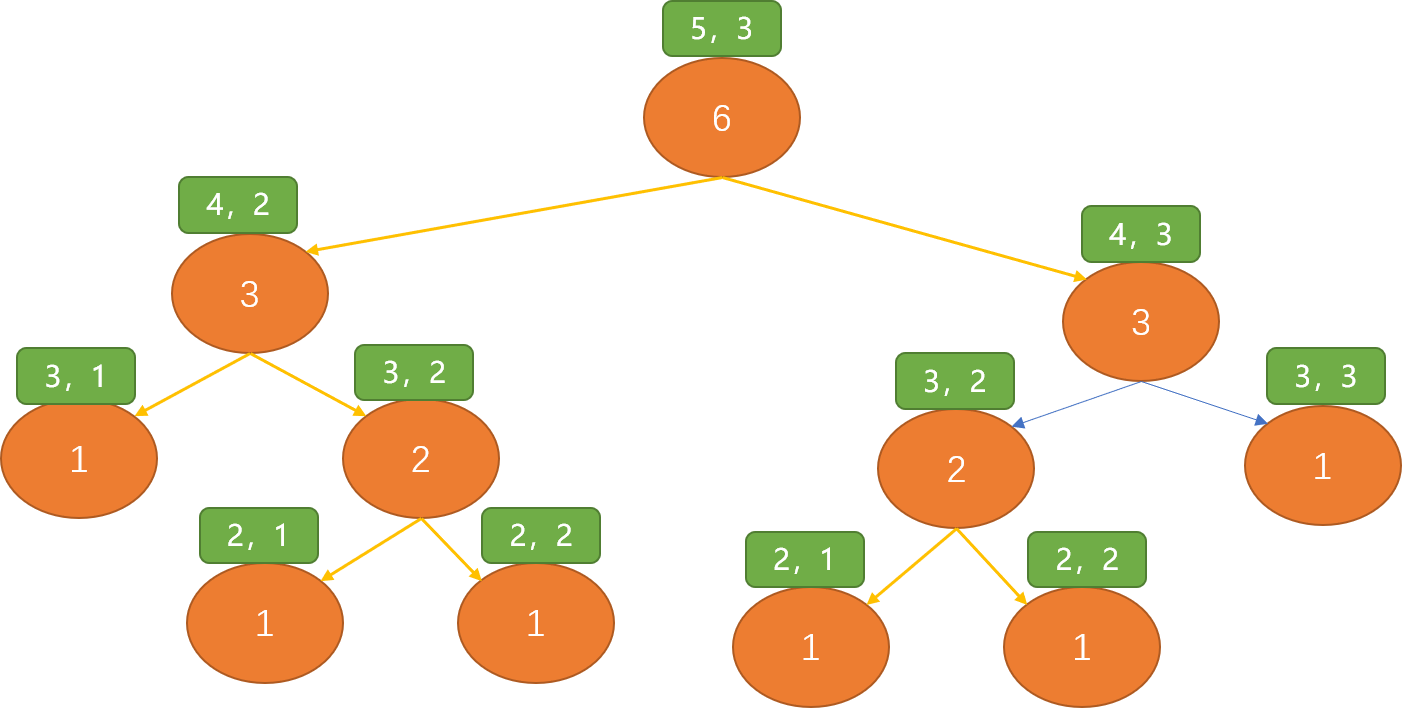# Python 基础知识自检，离深入掌握 Python 还有多远## 1. 模块化编程思想

Python 提供了大量的模块库，这些模块中有 Python 语言系统自带的、也有第三方提供的、也可以由开发者根据需要创建。

``````pip3 install 模块
``````

pip 或 pip3 是命令行程序。使用之前，需要进入 OS 的命令模式。windows 操作系统中使用 win+r 打开运行对话框，输入 cmd ，进入命令操作模式 。

``````Commands:
install                     安装模块
uninstall                   卸载模块
list                        列表出安装的模块
show                        显示安装模块的信息
check                       验证模块的依赖信息
config                      管理本地和全局配置
search                      从 PyPI (Python Package Index 是 Python 编程语言的软件存储库） 搜索模块
index                       使用模块的索引检查模块的合法性
``````

Python 语言系统中一个文件就是一个模块，一个模块中可以封装很多实用的功能代码，这些功能体可以以函数的形式存在，也可以以类的形式存存，模块具有完全的自我独立性。

## 2. 函数

Python 语言中，函数是一等公民。

``````def 函数名(函数参数)：
函数体
``````
• 定义函数，也就是创建一个函数必须使用 def 关键字。
• 函数名是函数的唯一标识符，也是使用函数的接口（入口）。
• 函数参数：用来接收调用者传过来的数据变量。也称为形式参数（占位符）。参数可以没有，也可以有多个。
• 函数体：函数提供的功能实现。

Python 函数的特点：

Python 既是面向对象编程语言，也是面向函数的编程语言。函数可以作为其它函数的参数，也可以作为其它函数的返回值。函数本质是一种数据类型

``````def han_shu():
print(1)
print(type(han_shu))
'''

<class 'function'>
'''
``````

1. 函数作为参数：
``````def a_han_shu(f):
f()
def b_han_shu():
print('Test')
# 函数作为参数不要有括号。
a_han_shu(b_han_shu)
'''

Test
'''
``````
1. 函数作为返回值：
``````def a_han_shu():
def b_han_shu():
print('Test')
return b_han_shu
# 返回的是对 b_han_shu 函数的引用
f=a_han_shu()
f()
'''

Test
'''
``````

1. 使用其它模块中的函数作参数：
``````import turtle
def forward(f):
f(200)
# turtle.forward 后面不能有括号
forward(turtle.forward)
'''

'''
``````
1. 类的方法本质还是函数
``````class Dog:
def __init__(self, name):
self.name = name

def run(self):
print(self.name,"在 run……")

def bark(self, f):
f()
print(self.name,"在 叫……")

d = Dog('小花')
d.bark(d.run)
'''

'''
``````

1. 位置传递参数：
``````def han_shu(num1, num2):
return num1 - num2

#如果想得到 10-4 相减的结果，调用时 10，4 的顺序不能颠倒。如果传递4，10 得到 4-10 结果
res = han_shu(10, 4)
print(res)
'''

6
'''
``````
1. 命名传递参数：
``````def han_shu(num1, num2):
return num1 - num2

#通过参数名传递数据
res = han_shu(num1=10,num2=4)
print(res)
# 传递参数时可以颠倒顺序
res = han_shu(num2=4,num1=10)
print(res)
'''

6
'''
``````
1. 默认值传递
``````def han_shu(num1, num2=4):
return num1 - num2

#通过参数名传递数据
res = han_shu(10)
print(res)
'''

6
'''
``````

1. 可变长度参数
``````def han_shu(*num):
return num
res = han_shu(10, 4,6,12)
print(res)
'''

(10, 4, 6, 12)
'''
``````

``````def han_shu(**num):
return num
res = han_shu(a=1,b=2,c=3)
print(res)
'''

{'a': 1, 'b': 2, 'c': 3}
'''
``````

• 没有函数名。
• 只能使用一次。
• 只能有一句函数体。
• 不能有 return 语句，默认返回唯一语句块的计算结果。
``````def han_shu():
return lambda num: num + 1
res = han_shu()
print(res(12))
'''

13
'''
``````

``````def han_shu():
def f(num):
return num + 1
return f
res = han_shu()
print(res(12))
'''

'''
``````

Python 中有很多内置函数、或称为系统函数，此类函数特点：可以直接使用。

input([x]) 交互式方式获取用户输入的数据 数据是字符串类型
print(x) 将 x 值输出到控制台 不指定输出内容时，输出换行
pow(x,y) x 的 y 次幂，相当于 x**y
round(x,[,n]) 对 x 四舍五入，保留 n 位小数点 不指定 n 时，不保留小数位
max(x1,x2,x3,……) 返回所数列中的最大值
min(x1,x2,x3,……) 返回数列中的最小值
sum(x1,x2,x3,……) 返回数列中所有数字相加之和 参数需是可迭代类型
len( ) 返回元组、列表、集合、字符串等容器对象的长度
range(start,end,step) 返回一个可迭代的对象 有index()、和count()方法
eval(x) 执行一个字符串表达式 可构建动态表达式
int(x) 将 x 转换成 int 类型数据 x 可以是字符串或浮点类型
float(x) 将 x 转换成 float 类型数据 可以是 int 或 str 类型
str(x) 将 x 转换成 str 类型
list(x) 将一个可迭代对象转换成列表
open() 打开一个文件
abs(x) 返回 x 的绝对值
type(x) 返回 x 的数据类型
ord(x) 返回字符串的 unicode 编码
chr(x) 返回 unicode 对应的字符串
sorted(x) 排序操作
tuple(x) 将可迭代对象转换成元组
set(x) 将可迭代对象转换成集合

## 3. 递归算法

1、递进过程：如有一个函数 a 。递归调用过程：第一次调用 a ===> 第二次调用 a ===>第三次调用 a => ……=>第 n 次调用 a。如果没有任何中止条件，则会无限制推进，导致内存耗尽。所以，递归必须有一个终结条件。

2、回溯过程：回溯过程是递进过程的逆过程。函数调用的特点是， a 调用 b 后，b 结束一定要返回到 a。在递归调用过程中，当第 n 次调用完成后，会进入第 n-1 次，再进入 n-2 次……一直回到第一次调用。

1. 递进过程：5！=5X4! ，如果求出 4! 则 5！也就可求出，而 4!=4X3!，问题变成求 3！的阶乘，而 3!=3X2!，问题变成求 2！，而 2！=2X1！，问题变成求 1!，而 1! 就是 1。到此递进过程可以终止。

2. 回溯过程：把 1! 的结果返回 2！,再把求得的 2！的结果返回求 3！，再把 3！的结果返回给求 4！，再把 4！的结果返回给 5！。最后得到结果。

``````def jc(num):
# 递归终止
if num == 1:
return 1
return num * jc(num - 1)
res = jc(5)
print(res)
``````1，1，2，3，5，8，13，21……``````def fb(pos):
if pos==1 or pos==2:
return 1
return fb(pos-1)+fb(pos-2)
res=fb(5)
print(res)
'''

5
'''
``````

``````#缓存器，位置作键，此位置的数字为值
cache = {}
def fb(pos):
if pos == 1 or pos == 2:
return 1
# 查看缓存中是否存在此位置已经计算出来的数字
if cache.get(pos) is not None:
return cache.get(pos)
# 缓存中没有，才递进
val = fb(pos - 2) + fb(pos - 1)
#得到值别忘记缓存
cache[pos] = val
return val
res = fb(5)
print(res)
'''

5
'''
````````````import time
cache = {}
def yh(row, col):
if row == 1 or col == 1 or row == col:
return 1
return yh(row - 1, col - 1) + yh(row - 1, col - 2)
s = time.process_time()
res = yh(25, 5)
e = time.process_time()
print(res)
print('所使用的时间：',e - s)
'''

5242885

'''
``````

``````import time
def yh(row, col):
if row == 1 or col == 1 or row == col:
return 1
if cache.get((row, col)) is not None:
return cache.get((row, col))
val = yh(row - 1, col - 1) + yh(row - 1, col - 2)
cache[(row, col)] = val
return val

s = time.process_time()
res = yh(25, 5)
e = time.process_time()
print(res)
print("使用时间:",e - s)
'''

5242885

'''
``````

## 4. 文件操作

w 可写，w+ 可读可写，当以 w 模式打开时，文件可以不存在，如果存在，文件中内容会被清除。

a 可追加写，a+ 可追加写，可读。当以 a 模式打开时，文件可以不存在，如果存在，文件中内容不会被清除。

``````with open("d:/temp.txt","r") as f :
# 读所有内容
# 读一行
# 读出所有行
``````

``````with open("d:/temp.txt","w") as f :
# 写入数据
f.write(1)
# 把列表数据写入
f.writelines([1,2])
# 使用 print 方法写入
print(123,34,sep=",",file=f)
``````